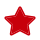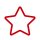Updated at: 2022-08-03 GMT+08:00

# Column Design

#### Selecting a Data Type

Comply with the following rules to improve query efficiency when you design columns:

• [Proposal] Use the most efficient data types allowed.

If all of the following number types provide the required service precision, they are recommended in descending order of priority: integer, floating point, and numeric.

• [Proposal] In tables that are logically related, columns having the same meaning should use the same data type.
• [Proposal] For string data, you are advised to use variable-length strings and specify the maximum length. To avoid truncation, ensure that the specified maximum length is greater than the maximum number of characters to be stored. You are not advised to use CHAR(n), BPCHAR(n), NCHAR(n), or CHARACTER(n), unless you know that the string length is fixed.

For details about string types, see Common String Types.

#### Common String Types

Every column requires a data type suitable for its data characteristics. The following table lists common string types in GaussDB(DWS).

Table 1 Common string types

Parameter

Description

Max. Storage Capacity

CHAR(n)

Fixed-length string, where n indicates the stored bytes. If the length of an input string is smaller than n, the string is automatically padded to n bytes using NULL characters.

10 MB

CHARACTER(n)

Fixed-length string, where n indicates the stored bytes. If the length of an input string is smaller than n, the string is automatically padded to n bytes using NULL characters.

10 MB

NCHAR(n)

Fixed-length string, where n indicates the stored bytes. If the length of an input string is smaller than n, the string is automatically padded to n bytes using NULL characters.

10 MB

BPCHAR(n)

Fixed-length string, where n indicates the stored bytes. If the length of an input string is smaller than n, the string is automatically padded to n bytes using NULL characters.

10 MB

VARCHAR(n)

Variable-length string, where n indicates the maximum number of bytes that can be stored.

10 MB

CHARACTER VARYING(n)

Variable-length string, where n indicates the maximum number of bytes that can be stored. This data type and VARCHAR(n) are different representations of the same data type.

10 MB

VARCHAR2(n)

Variable-length string, where n indicates the maximum number of bytes that can be stored. This data type is added to be compatible with the Oracle database, and its behavior is the same as that of VARCHAR(n).

10 MB

NVARCHAR2(n)

Variable-length string, where n indicates the maximum number of bytes that can be stored.

10 MB

TEXT

Variable-length string. Its maximum length is 8203 bytes less than 1 GB.

8203 bytes less than 1 GB